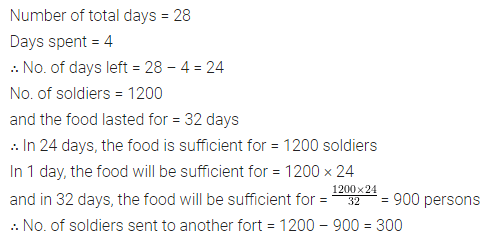# ML Aggarwal Class 8 Solutions for ICSE Maths Chapter 9 Direct and Inverse Variation Ex 9.2

## ML Aggarwal Class 8 Solutions for ICSE Maths Chapter 9 Direct and Inverse Variation Ex 9.2

Question 1.
Which of the following are in inverse variation?
(i) The number of students in a hostel and the consumption of food.
(ii) Time is taken by a train to cover a fixed distance and the speed of the train.
(iii) Area of land and its cost.
(iv) The number of people working and the time to complete the work.
(v) The quantity of rice and its cost.
Solution:Question 2.
Observe the following tables and find which pair of variables (here x and y) are in inverse variation: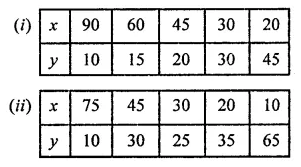Solution:Question 3.
Under the condition that the temperature remains constant, the volume of gas is inversely proportional to its pressure. If the volume of gas is 630 cubic centimetres at a pressure of 360 mm of mercury, then what will be the pressure of the gas, if its volume is 720 cubic centimetres at the same temperature?
Solution:Question 4.
A packet of sweets was distributed among 20 children and each of them received 4 sweets. How many sweets will each child get, if the number of children is reduced by 4?
Solution: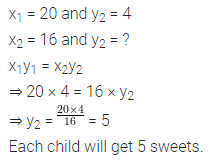Question 5.
Pooja has enough money to buy 36 oranges at the rate of ₹4.50 per orange. How many oranges she can buy if the price of each orange is increased by 90 paise?
Solution: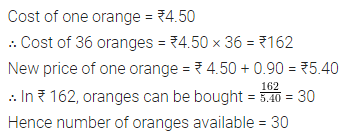Question 6.
It takes 8 days for 12 men to construct a wall. How many men should be put on the job if it is required to be constructed in 6 days.?
Solution: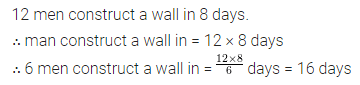Question 7.
Eight taps through which water flows at the same rate can fill a tank in 27 minutes. If two taps go out of order, how long will the remaining taps take to fill the tank?
Solution: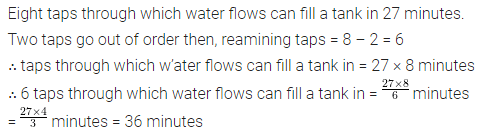Question 8.
A contractor undertook a contract to complete a part of a stadium in 9 months with a team of 560 persons. Later on, it was required to complete the job in 5 months. How many extra persons should he employ to complete the work?
Solution: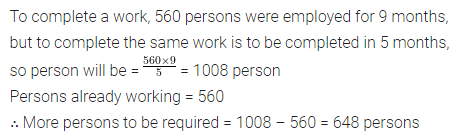Question 9.
A batch of bottles were packed in 30 boxes with 10 bottles in each box. If the same batch is packed using 12 bottles in each box, how many boxes would be filled?
Solution:Question 10.
Vandana takes 24 minutes to reach her school if she goes at a speed of 5 km/h. If she wants to reach school in 20 minutes, what should be her speed?
Solution: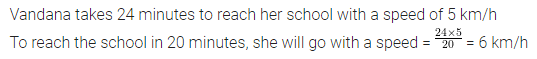Question 11.
A fort is provided with food for 80 soldiers to last for 60 days. Find how long would the food last if 20 additional soldiers join after 15 days.
Solution: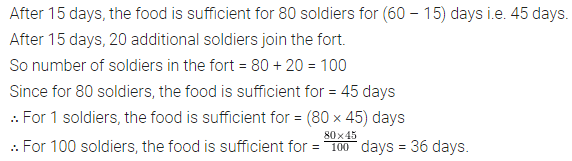Question 12.
1200 soldiers in a fort had enough food for 28 days. After 4 days, some soldiers were sent to another fort and thus, the food lasted for 32 more days. How many soldiers left the fort?
Solution: